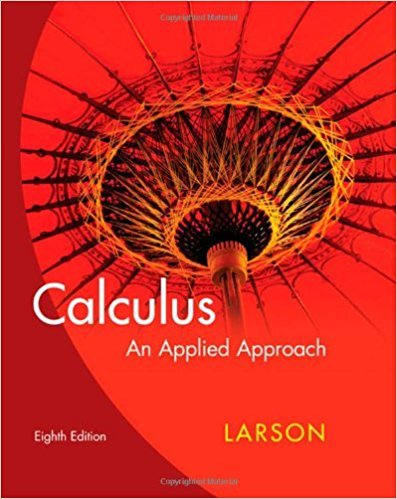×
×

# Solutions for Chapter 10: Series and Taylor Polynomials## Full solutions for Calculus: An Applied Approach | 8th Edition

ISBN: 9780618958252Solutions for Chapter 10: Series and Taylor Polynomials

Solutions for Chapter 10
4 5 0 393 Reviews
19
5
##### ISBN: 9780618958252

This expansive textbook survival guide covers the following chapters and their solutions. Since 112 problems in chapter 10: Series and Taylor Polynomials have been answered, more than 24021 students have viewed full step-by-step solutions from this chapter. Calculus: An Applied Approach was written by and is associated to the ISBN: 9780618958252. Chapter 10: Series and Taylor Polynomials includes 112 full step-by-step solutions. This textbook survival guide was created for the textbook: Calculus: An Applied Approach , edition: 8.

Key Calculus Terms and definitions covered in this textbook
• Categorical variable

In statistics, a nonnumerical variable such as gender or hair color. Numerical variables like zip codes, in which the numbers have no quantitative significance, are also considered to be categorical.

• Coordinate plane

See Cartesian coordinate system.

• Distributive property

a(b + c) = ab + ac and related properties

• Histogram

A graph that visually represents the information in a frequency table using rectangular areas proportional to the frequencies.

• Independent variable

Variable representing the domain value of a function (usually x).

• Infinite sequence

A function whose domain is the set of all natural numbers.

• Initial value of a function

ƒ 0.

• Law of cosines

a2 = b2 + c2 - 2bc cos A, b2 = a2 + c2 - 2ac cos B, c2 = a2 + b2 - 2ab cos C

• Midpoint (in Cartesian space)

For the line segment with endpoints (x 1, y1, z 1) and (x2, y2, z2), ax 1 + x 22 ,y1 + y22 ,z 1 + z 22 b

• Partial fraction decomposition

See Partial fractions.

• Polar coordinates

The numbers (r, ?) that determine a point’s location in a polar coordinate system. The number r is the directed distance and ? is the directed angle

• Position vector of the point (a, b)

The vector <a,b>.

• Richter scale

A logarithmic scale used in measuring the intensity of an earthquake.

• Second-degree equation in two variables

Ax 2 + Bxy + Cy2 + Dx + Ey + F = 0, where A, B, and C are not all zero.

• Solution set of an inequality

The set of all solutions of an inequality

• Speed

The magnitude of the velocity vector, given by distance/time.

• Standard form of a polynomial function

ƒ(x) = an x n + an-1x n-1 + Á + a1x + a0

• Sum of a finite arithmetic series

Sn = na a1 + a2 2 b = n 2 32a1 + 1n - 12d4,

• Term of a polynomial (function)

An expression of the form anxn in a polynomial (function).

• z-coordinate

The directed distance from the xy-plane to a point in space, or the third number in an ordered triple.

×